## Selina Concise Mathematics Class 7 ICSE Solutions Chapter 16 Pythagoras Theorem

Selina Publishers Concise Maths Class 7 ICSE Solutions Chapter 16 Pythagoras Theorem

APlusTopper.com provides step by step solutions for Selina Concise ICSE Solutions for Class 7 Mathematics. You can download the Selina Concise Mathematics ICSE Solutions for Class 7 with Free PDF download option. Selina Publishers Concise Mathematics for Class 7 ICSE Solutions all questions are solved and explained by expert mathematic teachers as per ICSE board guidelines.

### Pythagoras Theorem Exercise 16 – Selina Concise Mathematics Class 7 ICSE Solutions

Question 1.
Triangle ABC is right-angled at vertex A. Calculate the length of BC, if AB = 18 cm and AC = 24 cm.

Solution:
Given : ∆ABC right angled at A and AB = 18 cm, AC = 24 cm.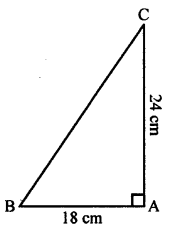To find : Length of BC.
According to Pythagoras Theorem,
BC2 = AB2 + AC2
= 182 + 242 = 324 + 576 = 900
∴BC = $$\sqrt { 900 }$$ = $$\sqrt { 30 x 30 }$$= 30 cm

Question 2.
Triangle XYZ is right-angled at vertex Z. Calculate the length of YZ, if XY = 13 cm and XZ = 12 cm.

Solution:
Given : ∆XYZ right angled at Z and XY = 13 cm, XZ = 12 cm.
To find : Length of YZ.
According to Pythagoras Theorem,
XY2 = XZ2 + YZ2
132 = 122 + YZ2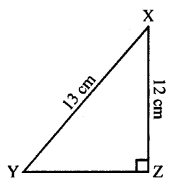169= 144 +YZ2
169- 144 = YZ2
25 = YZ2
∴YZ = $$\sqrt { 25 }$$cm $$\sqrt { 5×5 }$$ = 5 cm

Question 3.
Triangle PQR is right-angled at vertex R. Calculate the length of PR, if:
PQ = 34 cm and QR = 33.6 cm.

Solution:
Given : ∆PQR right angled at R and PQ = 34 cm, QR = 33.6 cm.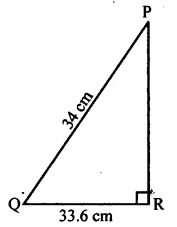To find : Length of PR.
According to Pythagoras Theorem,
PR2 + QR2 = PQ2
PR2 + 33.62 = 342
PR2+ 1128.96= 1156
PR2 = 1156- 1128.96
∴ PR = $$\sqrt { 27.04 }$$ = 5.2 cm

Question 4.
The sides of a certain triangle are given below. Find, which of them is right-triangle
(i) 16 cm, 20 cm and 12 cm
(ii) 6 m, 9 m and 13 m

Solution:
(i) 16 cm, 20 cm and 12 cm
The given triangle will be a right-angled triangle if square of its largest side is equal to the sum of the squares on the other two sides.
i.e., If (20)2 = (16)2 = (12)2
(20)2 = (16)2 + (12)2
400 = 256 + 144
400 = 400
So, the given triangle is right angled.
(ii) 6 m, 9 m and 13 m
The given triangle will be a right-angled triangle if square of its largest side is equal to the sum of the squares on the other two sides.
i.e., If (13)2 = (9)2 + (6)2
169 = 81+36 169 ≠ 117
So, the given triangle is not right angled.

Question 5.
In the given figure, angle BAC = 90°, AC = 400 m and AB = 300 m. Find the length of BC.Solution:
AC = 400 m
AB = 300 m
BC = ?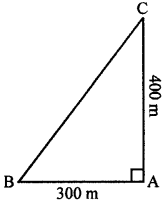According to Pythagoras Theorem,
BC2 = AB2 + AC2
BC2 = (300)2 + (400)2
BC2 = 90000 + 160000
BC2 = 250000
BC = $$\sqrt { 250000 }$$= 500 m

Question 6.
In the given figure, angle ACP = ∠BDP = 90°, AC = 12 m, BD = 9 m and PA= PB = 15 m. Find:

(i) CP
(ii) PD
(iii) CD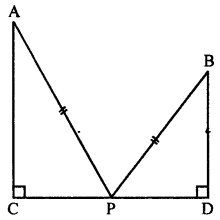Solution:
Given : AC = 12 m
BD = 9 m
PA = PB= 15 m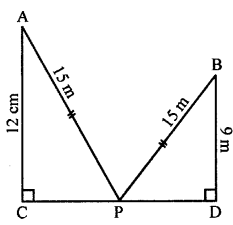(i) In right angle triangle ACP
(AP)2 = (AC)2 + (CP)2
152 = 122 + CP2
225 = 144 + CP2
225 – 144 = CP2
81 =CP
$$\sqrt { 81 }$$ =CP
∴ CP = 9 m
(ii) In right angle triangle BPD
(PB)2 = (BD)2 + (PD)2
(15)2 = (9)2 + PD2
225 = 81 + PD2
225-81 = PD2
144 = PD2
$$\sqrt { 144 }$$=PD                                               ‘
∴ PD = 12 m
(iii) CP = 9 m
PD = 12 m
∴ CD = CP + PD
= 9+ 12 = 21 m

Question 7.
In triangle PQR, angle Q = 90°, find :
(i) PR, if PQ = 8 cm and QR = 6 cm
(ii) PQ, if PR = 34 cm and QR = 30 cm

Solution:
(i) Given:
PQ = 8 cm
QR = 6 cm
PR = ?
∠PQR = 90°According to Pythagoras Theorem,
(PR)2 = (PQ)2 + (QR)2
PR2 = 82 + 62
PR2 = 64 + 36
PR2 = 100
∴ PR = $$\sqrt { 100 }$$= 10 cm
(ii) Given :
PR = 34 cm
QR = 30 cm
PQ = ?
∠PQR = 90°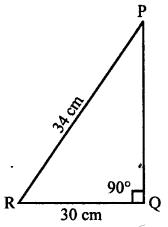According to Pythagoras Theorem,
(PR)2 = (PQ)2 + (QR)2
(34)2 = PQ2 + (30)2
1156 = PQ2 + 900
1156-900 = PQ2
256 = PQ2
∴ PQ = 16 cm

Question 8.
Show that the triangle ABC is a right-angled triangle; if:
AB = 9 cm, BC = 40 cm and AC = 41 cm

Solution:
AB = 9 cm
CB = 40 cm
AC = 41 cm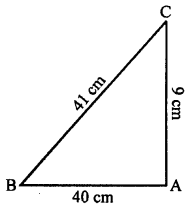The given triangle will be a right angled triangle if square of its largest side is equal to the sum of the squares on the other two sides.
According to Pythagoras Theorem,
(AC)2 = (BC)2 + (AB)2
(41)2 = (40)2 + (9)2
1681 = 1600 + 81
1681 = 1681
Hence, it is a right-angled triangle ABC.

Question 9.
In the given figure, angle ACB = 90° = angle ACD. If AB = 10 m, BC = 6 cm and AD = 17 cm, find :
(i) AC
(ii) CD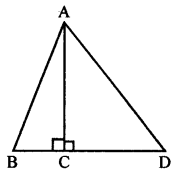Solution:
Given:
∆ABD
∠ACB = ∠ACD = 90°
and AB = 10 cm, BC = 6 cm and AD = 17 cmTo find:
(i) Length of AC
(ii) Length of CD
Proof:
(i) In right-angled triangle ABC
BC = 6 cm, AB = 110 cm
According to Pythagoras Theorem,
AB2 = AC2 + BC2
(10)2 = (AC)2 + (6)2
100 = (AC)2 + 36
AC2 = 100-36 = 64 cm
AC2 = 64 cm
∴ AC = $$\sqrt { 8×8 }$$ = 8 cm
(ii) In right-angle triangle ACD
AD = 17 cm, AC = 8 cm
According to Pythagoras Theorem,
(17)2 = (8)2 + (CD)2
289 – 64 = CD2
225 = CD2
CD =$$\sqrt { 15×15 }$$ = 15 cm

Question 10.
In the given figure, angle ADB = 90°, AC = AB = 26 cm and BD = DC. If the length of AD = 24 cm; find the length of BC.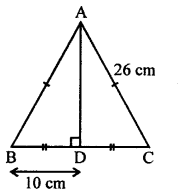Solution:
Given:
∆ABC
∠ADB = 90° and AC = AB = 26 cm
To find : Length of BC In right angled ∆ADC
AB = 26 cm, AD = 24 cm
According to Pythagoras Theorem,
(26)2 = (24)2 + (DC)2
676 = 576 + (DC)2
⇒ (DC)2 = 100
⇒ DC = $$\sqrt { 100 }$$ = 10 cm
∴ Length of BC = BD + DC
= 10 + 10 = 20 cm

Question 11.
In the given figure, AD = 13 cm, BC = 12 cm, AB = 3 cm and angle ACD = angle ABC = 90°. Find the length of DC.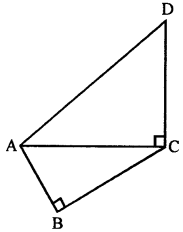Solution:
Given :
∆ACD = ∠ABC = 90°
and AD = 13 cm, BC = 12 cm, AB = 3 cm
To find : Length of DC.(i)In right angled ∆ABC
AB = 3 cm, BC = 12 cm
According to Pythagoras Theorem,
(AC)2 = (AB)2 + (BC)2
(AC)2 = (3)2 + (12)2
(AC) = $$\sqrt { 9+144 }$$ = $$\sqrt { 153 }$$ cm

(ii) In right angled triangle ACD
AD = 13 cm, AC =$$\sqrt { 153 }$$
According to Pythagoras Theorem,
DC2 = AB2-AC2
DC2= 169-153
DC = $$\sqrt { 16 }$$ = 4 cm
∴ Length of DC is 4 cm

Question 12.
A ladder, 6.5 m long, rests against a vertical wall. Ifthe foot of the ladcler is 2.5 m from the foot of the wall, find upto how much height does the ladder reach?

Solution:
Given :
Length of ladder = 6.5 m
Length of foot of the wall = 2.5 m
To find : Height AC According to Pythagoras Theorem,
(BC)2 = (AB)2 + (AC)2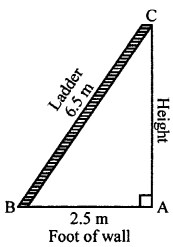(6.5)2 = (2.5)2 + (AC)2
42.25 = 6.25 + AC2
AC2 = 42.25 – 6.25 = 36 m
AC = $$\sqrt { 6×6 }$$ = 6 m
∴ Height of wall = 6 m

Question 13.
A boy first goes 5 m due north and then 12 m due east. Find the distance between the initial and the final position of the boy.

Solution:
Given : Direction of north = 5 m i.e. AC Direction of east = 12 m i.e. AB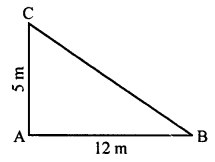To find: BC
According to Pythagoras Theorem,
In right angled AABC
(BC)2 = (AC)2 + (AB)2
(BC)2 = (5)2 + (12)2
(BC)2 = 25 + 144
(BC)2 = 25 + 144
(BC)2= 169
∴ BC = $$\sqrt { 169 }$$ = $$\sqrt { 13×13 }$$ = 13 m

Question 14.
Use the information given in the figure to find the length AD.Solution:
Given :
AB = 20 cm
∴AO =$$\frac { AB }{ 2 }$$ = $$\frac { 20 }{ 2 }$$ =10cm
BC = OD = 24 cm
To find : Length of AD
In right angled triangle
AOD (AD)2 = (AO)2 + (OD)2
∴ AD =  $$\sqrt { 26×26 }$$